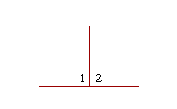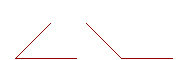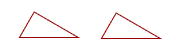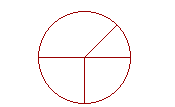P l a n e  G e o m e t r y

An Adventure in Language and Logic

based on# First Principles

Definitions

IT IS NOT POSSIBLE to prove every statement; we saw that in the Introduction. Nevertheless, we should prove as many statements as possible. Which is to say, the statements we do not prove should be as few as possible. They are called the First Principles. They fall into three categories: Definitions, Postulates, and Axioms or Common Notions. We will follow each with a brief commentary.

## Definitions11.  An angle is the inclination to one another of two straight lines that meet.

12.  The point at which two lines meet is called the vertex of the angle.13.  If a straight line that stands on another straight line makes the adjacent angles equal,
then each of those angles is called a right angle;
and the straight line that stands on the other is called a perpendicular to it.14.  An acute angle is less than a right angle. An obtuse angle is greater than a right angle.

 5.  Angles are complementary (or complements of one another) if together they equal a right angle. Angles are supplementary (or supplements of one another) if together they equal two right angles.16.  Rectilinear figures are figures bounded by straight lines.  A triangle is bounded by three straight lines, a quadrilateral by four, and a polygon by more than four straight lines.17.  A square is a quadrilateral in which all the sides are equal, and all the angles are right angles.18.  A regular polygon has equal sides and equal angles.

19.  An equilateral triangle has three equal sides.  An isosceles triangle has two equal sides. A scalene triangle has three unequal sides.10.  The vertex angle of a triangle is the angle opposite the base.

11.  The height of a triangle is the straight line drawn from the vertex perpendicular to the base.

12.  A right triangle is a triangle that has a right angle.

13.  Figures are congruent when,if one of them were placed on the other, they would exactly coincide.  Congruent figures are thus equal to one another in all respects.

 14.  Parallel lines are straight lines that are in the same plane and do not meet, no matter how far extended in either direction.15.  A parallelogram is a quadrilateral whose opposite sides are parallel

16.  Acircle is a plane figure bounded by one line, called the circumference, such that all straight lines drawn from a certain point within the figure to the circumference, are equal to one another.

17.  And that point is called the center of the circle.

18.  A diameter of a circle is a straight line through the center and terminating in both directions on the circumference.  A straight line from the center to the circumference is called a radius; plural, radii.

## Postulates

1.  Grant the following:

1.  To draw a straight line from any point to any point.

2.  To extend a straight line for as far as we please in a straight line.

3.  To draw a circle whose center is the extremity of any straight line, and whose radius is the straight line itself.

4.  All right angles are equal to one another.

5.  If a straight line that meets two straight lines makes the interior angles on the same side less than two right angles, then those two straight lines, if extended, will meet on that same side.(That is, if angles 1 and 2 together are less than two right angles, then the straight lines AB, CD, if extended far enough, will meet on that same side; which is to say, AB, CD are not parallel.)

## Axioms or Common Notions

1.  Things equal to the same thing are equal to one another.

2.  If equals are added to equals, the wholes will be equal.3.  If equals are taken from equals, what remains will be equal.4.  Things that coincide with one another are equal to one another.

5.  The whole is greater than the part.

6.  Equal magnitudes have equal parts; equal halves, equal thirds,

2.  and so on.Commentary on the Definitions

A definition clarifies the idea of what is being defined, and gives it a name. What has that name obviously exists as an idea, for we have understood the definition; or at least, we should. But to say that something exists for mathematics, we must mean that it exists not merely as an idea. We must mean that we can represent in the physical world. A definition does not assume that; it simply states what the word means.

And so an "equilateral triangle" is defined. But the very first proposition presents the logical steps that allow us to construct a figure that satisfies the definition. We can then say that an equilateral triangle has its mathematical existence. The definition of an equilateral triangle describes something we can actually witness and draw.

Again, a figure is an idea. Its boundary—a line—is the idea of length only. But what we draw obviously has width. Therefore what we draw symbolizes or represents the idea. In fact, we say, "Let AB be the given straight line." We are asked to let AB represent that idea.

With any definition, then, we must either postulate the possibility of drawing what has that name (that is done in the case of a circle, Postulate 3), or we must prove it, as we do with an equilateral triangle.

By maintaining the logical separation of a definition and its physical representation, mathematics becomes a science in the same way that physics is a science. Physics must show that the things of which it speaks—"electrons," "protons," "neutrinos"—actually exist. And physics does that by showing that it is possible to experience them.  It was geometry that led the way. Geometry was the first science.  By requiring that a definition does not assert its physical existence, mathematics avoids dealing in fantasies and the possibility of contradictions. For example, by a "hemigon" I mean a rectilineal figure that has half as many sides as angles.  Do you understand?  Good.

A definition is reversible. That means that when the conditions of the definition are satisfied, then not only may we use that word. Conversely, if we use that word, that implies those conditions have been satisfied. A definition is equivalent to an if and only if sentence.

Note that the definition of a right angle says nothing about measurement, about 90°.  Plane geometry is not the study of how to apply arithmetic to figures. In geometry we are concerned only with what we can see and reason directly, not through computation.  A most basic form of knowledge is that two magnitudes are simply equal—not that they are both 90° or 9 meters.

How can we know when things are equal?  That is one of the main questions of geometry.  The definition (and existence) of a circle provides our first way of knowing that two straight lines could be equal. Because if we know that a figure is a circle, then we would know that any two radii are equal. (Definition 16.)

We have not formally defined a point, although Euclid does. "A point is that which has no part." That is, it is indivisible. Most significantly, Euclid adds, "The extremities of a line are points." Thus when a line exists—when it has been drawn, its endpoints also exist. And we have not defined a "line," although again Euclid does. "A line is length without breadth." Euclid defines them because they are rudimentary ideas in geometry. But since there is never occasion to prove that something is a point or a line, a definition of one is not logically required.

And so we may say that all definitions are technical, in that they define a necessary term of the science. The definitions of a radius, the vertex of an angle, and a regular polygon are technical.  A definition is also functional when we must satisfy it to prove a theorem or a problem. The definitions of a right angle, an isosceles triangle, and a square are functional—because we will have occasion to prove that something is a right angle, an isosceles triangle, and a square. We will never have occasion to prove that something is the vertex of an angle.

Commentary on the Postulates

We require that the figures of geometry—the triangles, squares, circles—be more than mental objects. We must be able to draw them; to represent them in the physical world. The fact that we can draw a figure permits us to say not only that it exists as an idea. But it exists logically.

The first three Postulates narrowly set down what we are permitted to draw. Everything else we must prove. Each of those Postulates is therefore a "problem"—a construction—that we are asked to consider solved:  "Grant the following."

The instruments of construction are straightedge and compassPostulate 1, in effect, asks us to grant that what we draw with a straightedge is a straight line.  Postulate 3 asks us to grant that the figure we draw with a compass is a circle. And so we will then have an actual figure that refers to the idea of a circle, rather than just the word "circle."

As for Postulate 5, we will have more to say about it when we come to Proposition 29.

Note, finally, that the word all, as in "all right angles" or "all straight lines," refer to all that exist, that is, all that we have actually drawn. Geometry—at any rate Euclid's—is never just in our mind.

Commentary on the Axioms or Common Notions

The distinction between a postulate and an axiom is that a postulate is about the specific subject at hand, in this case, geometry; while an axiom is a statement we acknowledge to be more generally true; it is in fact a common notion.  Yet each has the same logical function, which is to authorize statements in the proofs that follow.

Each of the Axioms, as well as Postulate 4, gives a criterion for things being equal (or, Axiom 5, unequal).

Implicit in these Axioms is our very understanding of equal versus unequal, which is:  Two magnitudes of the same kind are either equal or one of them is greater.

So, these Axioms, together with the Definitions and Postulates, are the first principles from which our theory of figures will be deduced.

Please "turn" the page and do some Problems.

or

Continue on to Proposition 1.

Please make a donation to keep TheMathPage online.
Even \$1 will help.